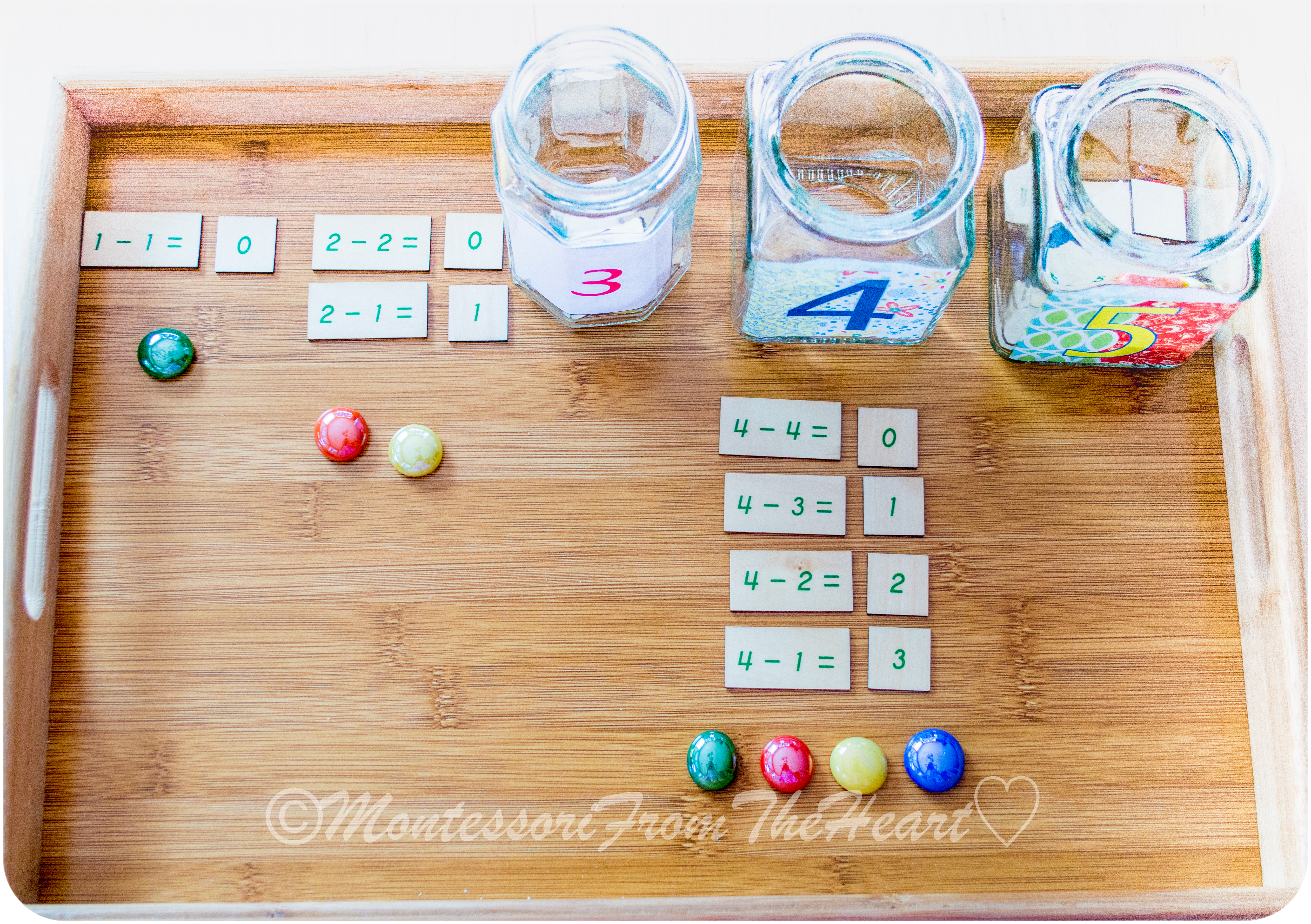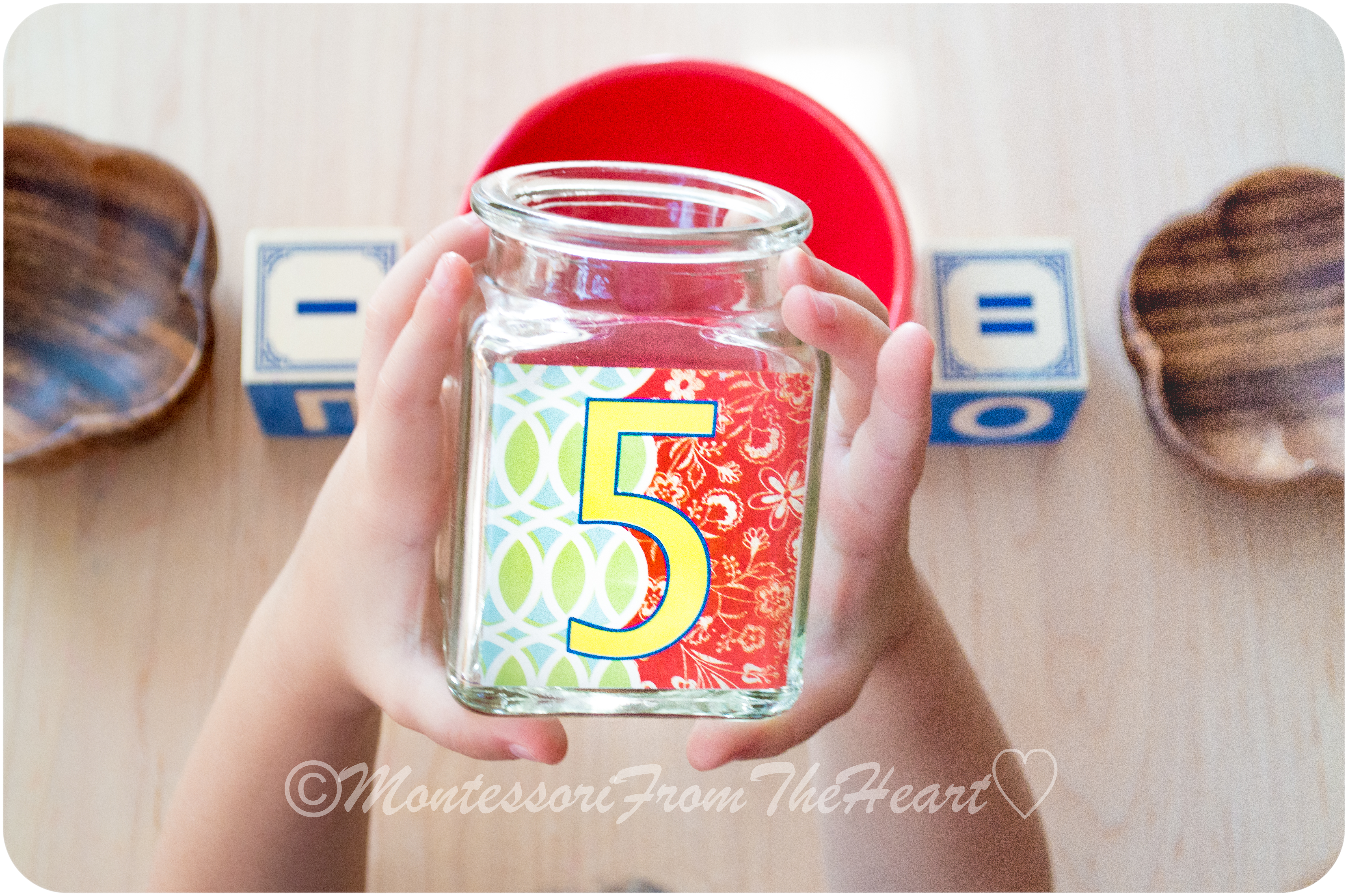This DIY lesson on Beginning Subtraction uses materials you probably have at your home. The lesson should be presented to a child who already understands the concept of numbers at least till ten and the concept of addition.

First, you need to make "Number-Jars" – see how below:For your "Number-Jars" you can use spice jars, jam jars or any other shallow dish for each number (lids are not necessary). So, for example, the "Number -4-Jar" must have the proper number of the following materials since the purpose of every Montessori lesson is to have a control of error:

• equations (see picture above) – so the "Number -4" Jar will have four equations (unless you would like to add an equation "minus zero"); you can use colored cardstock (buy here) and yourself write down the equations and then cut them out;  we are using wooden Montessori equations (similar here); alternately you can use these wooden numbers to "make" an equation;
• separate answers for each equation;
• the same amount of marbles as that Number-Jar, so in "Number -4" Jar, you will have four marbles;For numbers 1-jar and 2-jar, I am using shallow dishes since number "1-jar" will have just one equation with one answer and one marble, and number "2-jar" will have two equations, two answers, and two marbles.Adrian (3.5 years old) is working with the "Number-5-Jar."The equations should be positioned on one side of the work and the answers on the other side.